This resource function is obsolete. Use the paclet Wolfram/QuantumFramework instead.

Function Repository Resource:

# QuantumMeasurement

Represent the outcomes of a quantum measurement

Contributed by: Jonathan Gorard and Taufiq Murtadho
 ResourceFunction["QuantumMeasurement"][<|o1→p1,o2→p2,…|>,{QuantumDiscreteState[…],QuantumDiscreteState[…],…}] represents the result of a quantum measurement with possible outcomes oi and associated probabilities pi, with a list of QuantumDiscreteState objects representing the possible quantum states of the system following the measurement operation.

## Details

ResourceFunction["QuantumMeasurement"] shares many properties in common with CategoricalDistribution.
The Association <|o1p1,o2p2,|> has keys given by named measurement outcomes oi and values given by probabilities pi.
ResourceFunction["QuantumMeasurement"][]["prop"] gives the property "prop" of the specified quantum measurement. Possible properties include:
 "Distribution" CategoricalDistribution object representing the measurement outcome(s) "Outcomes" list of possible measurement outcomes "Probabilities" association of measurement outcomes and their respective probabilities "ProbabilitiesList" list of measurement probabilities "ProbabilityTable" measurement outcomes and their probabilities in a Dataset "ProbabilityArray" measurement probabilities in a SparseArray "ProbabilityPlot" visualization of the measurement probability function "TopProbabilities" list of measurement outcomes with the highest probabilities "TopProbabilities"→n list of top n measurement outcomes with the highest probabilities "Entropy" exact entropy of the measurement distribution "NEntropy" numerical approximation of entropy of the measurement distribution "SimulatedMeasurement" outcome of one simulated measurement {"SimulatedMeasurement",n} list of outcomes from n simulated measurements "Mean" (symbolic) mean of all measurement outcomes "States" list of possible QuantumDiscreteState objects following measurement "StateAssociation" association of measurement outcomes and their respective QuantumDiscreteState objects "StateProbabilities" association of QuantumDiscreteState objects and their respective probabilities "StateProbabilityTable" QuantumDiscreteState objects and their probabilities in a Dataset "TopStateProbabilities" list of QuantumDiscreteState objects with the highest probabilities "TopStateProbabilities"→n list of top n QuantumDiscreteState objects with the highest probabilities "SimulatedStateMeasurement" QuantumDiscreteState object yielded by one simulated measurement {"SimulatedStateMeasurement",n} list of QuantumDiscreteState objects yielded by n simulated measurements "MeanState" (symbolic) mean of all QuantumDiscreteState objects "Properties" list of all property names

## Examples

### Basic Examples (3)

Define a QuantumMeasurement object for a two-level quantum system with two possible measurement outcomes:

 In:=Out=Return the associated CategoricalDistribution object:

 In:=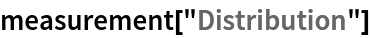Out=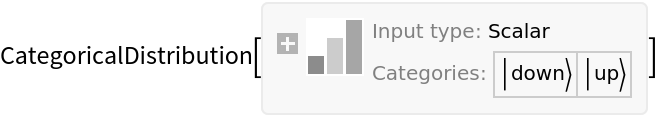Return the list possible QuantumDiscreteState objects following measurement:

 In:=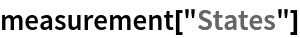Out=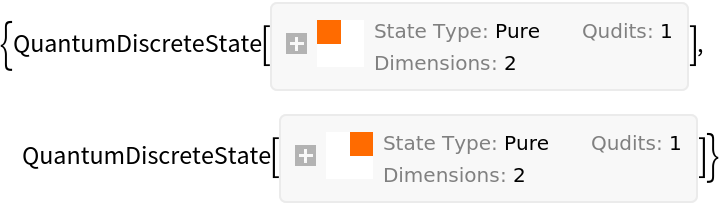Conduct a simulated measurement:

 In:=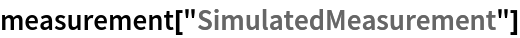Out=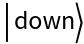Conduct 100 simulated measurements:

 In:=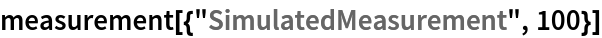Out=QuantumMeasurement automatically normalizes the input probabilities:

 In:=Out=Return the association of measurement outcomes and their respective probabilities:

 In:=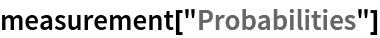Out=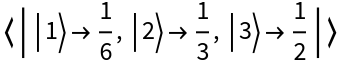Return the association of measurement outcomes and their respective QuantumDiscreteState objects:

 In:=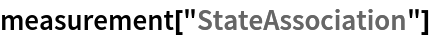Out=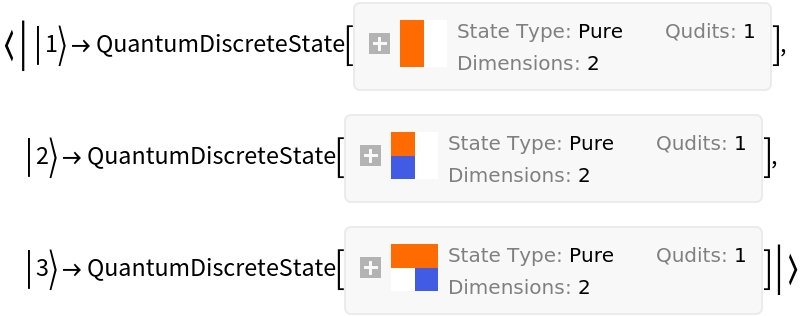Compute the QuantumDiscreteState object yielded by a simulated measurement:

 In:=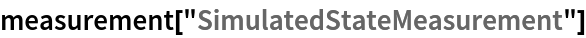Out=Compute the list of QuantumDiscreteState objects yielded by 10 simulated measurements:

 In:=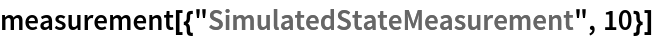Out=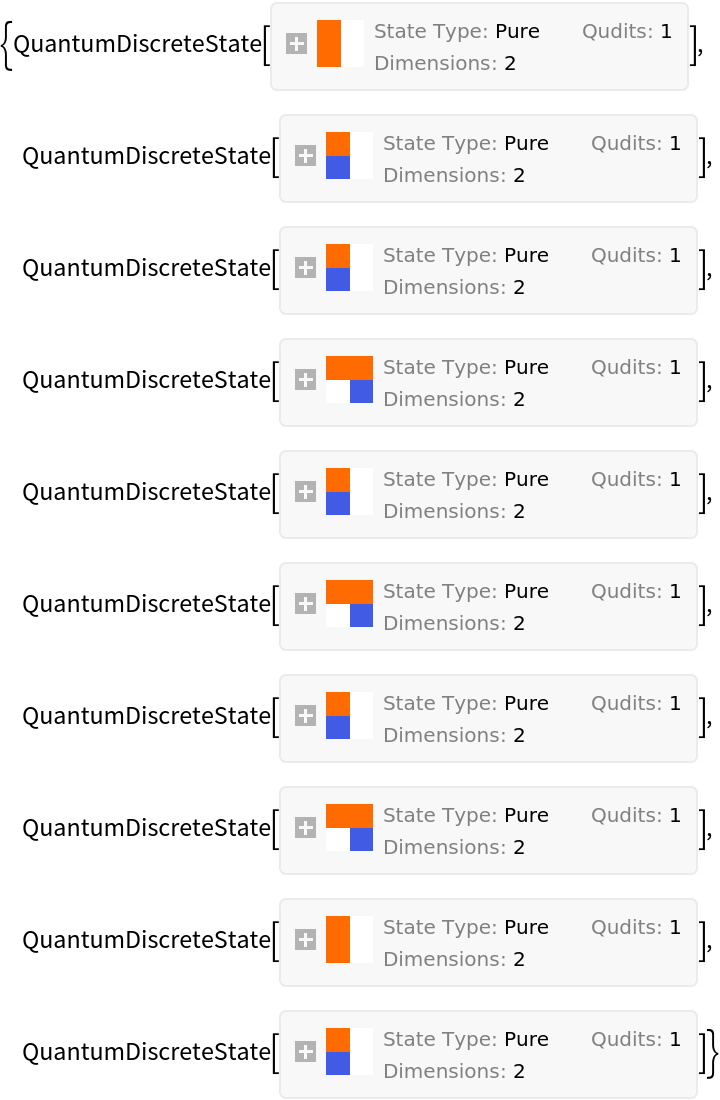Compute the exact entropy of the measurement distribution:

 In:=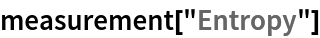Out=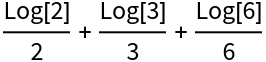Compute the approximate (numerical) entropy of the measurement distribution:

 In:=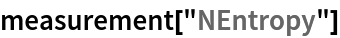Out=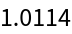Define a QuantumMeasurement object for a three-level quantum system with four possible measurement outcomes:

 In:=Out=Return the association of measurement outcomes and their respective probabilities:

 In:=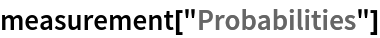Out=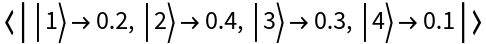Return the association of QuantumDiscreteState objects and their respective probabilities:

 In:=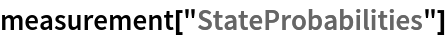Out=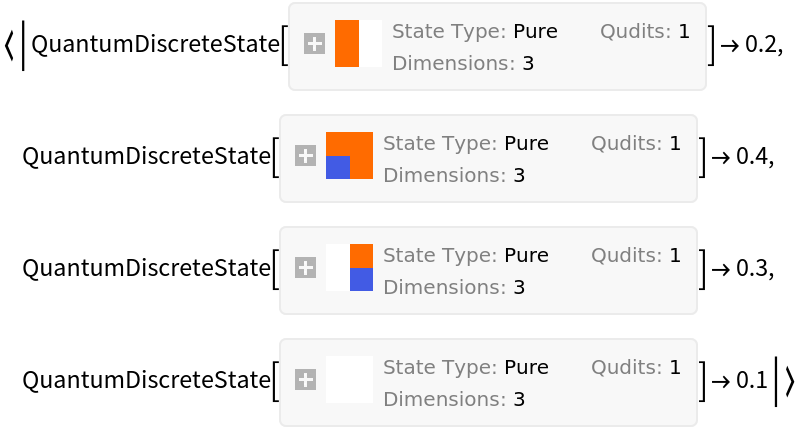### Scope (24)

View a list of properties that can be extracted from a QuantumMeasurement object:

 In:=Out=In:=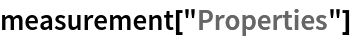Out=Return the CategoricalDistribution object representing the measurement outcomes:

 In:=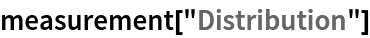Out=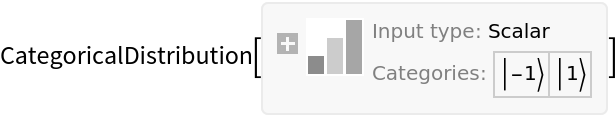Return the list of possible measurement outcomes:

 In:=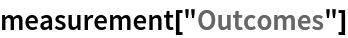Out=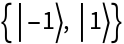Return the association of measurement outcomes and their respective probabilities:

 In:=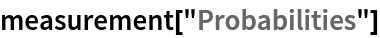Out=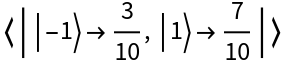Return the list of measurement probabilities:

 In:=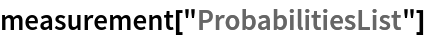Out=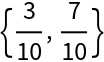Return the measurement outcomes and their probabilities in a Dataset:

 In:=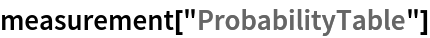Out=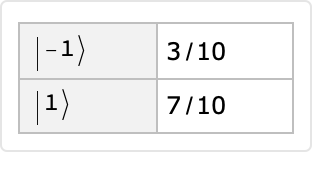Return the measurement probabilities in a SparseArray:

 In:=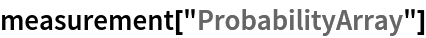Out=Visualize the measurement probability function:

 In:=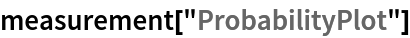Out=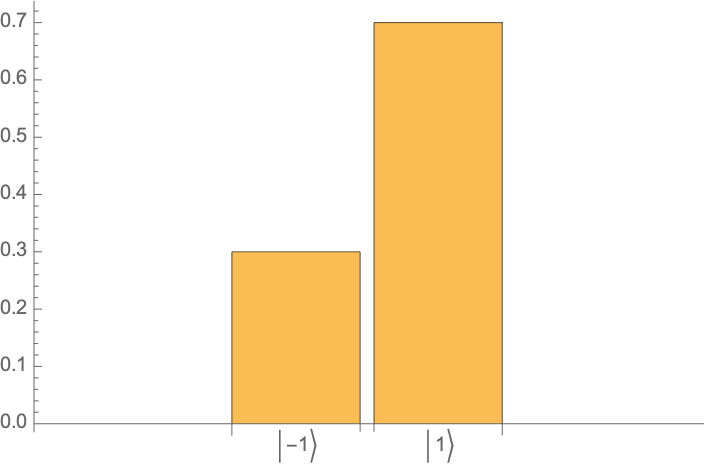Return the list of measurement outcomes with the highest probabilities:

 In:=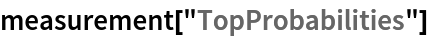Out=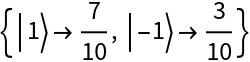Return the list of 1 top measurement outcomes with the highest probabilities:

 In:=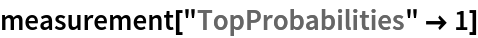Out=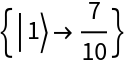Return the exact entropy of the measurement distribution:

 In:=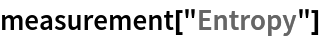Out=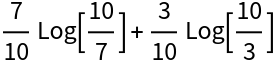Return the approximate (numerical) entropy of the measurement distribution:

 In:=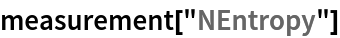Out=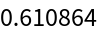Return the outcome of one simulated measurement:

 In:=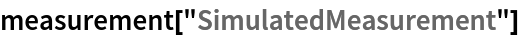Out=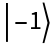Return the list of outcomes from 100 simulated measurements:

 In:=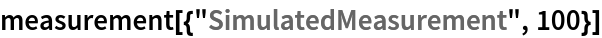Out=Return the symbolic mean of all measurement outcomes:

 In:=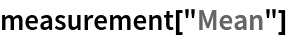Out=Return the list of possible QuantumDiscreteState objects following measurement:

 In:=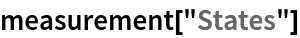Out=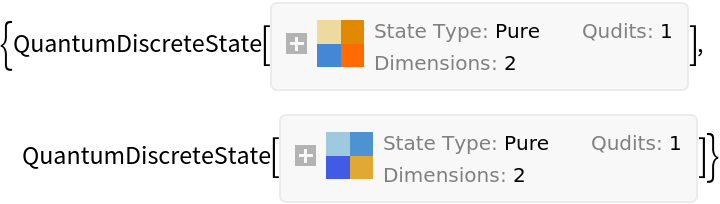Return the association of measurement outcomes and their respective QuantumDiscreteState objects:

 In:=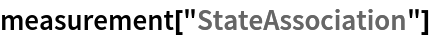Out=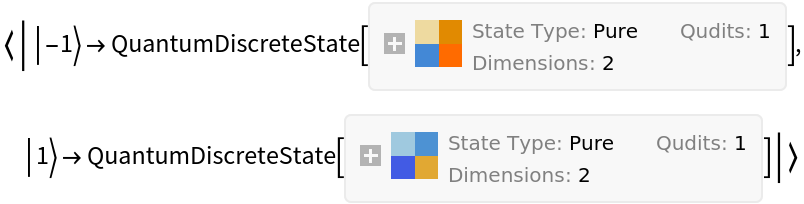Return the association of QuantumDiscreteState objects and their respective probabilities:

 In:=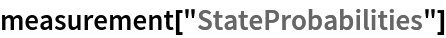Out=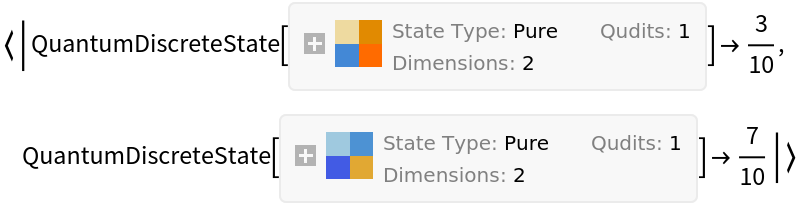Return the QuantumDiscreteState objects and their probabilities in a Dataset:

 In:=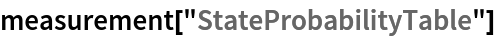Out=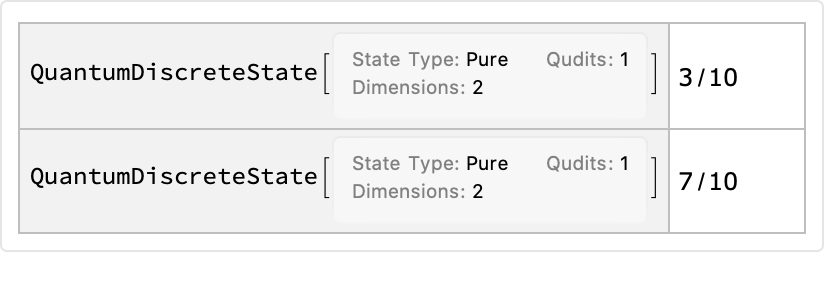Return the list of QuantumDiscreteState objects with the highest probabilities:

 In:=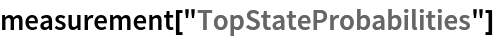Out=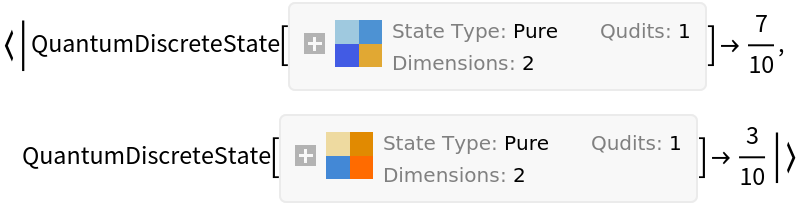Return the list of 1 top QuantumDiscreteState objects with the highest probabilities:

 In:=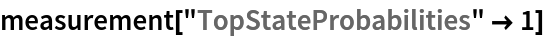Out=Return the QuantumDiscreteState object yielded by one simulated measurement:

 In:=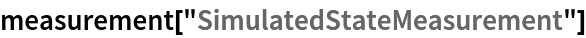Out=Return the list of QuantumDiscreteState objects yielded by 10 simulated measurements:

 In:=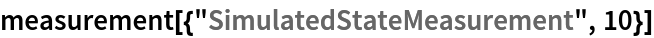Out=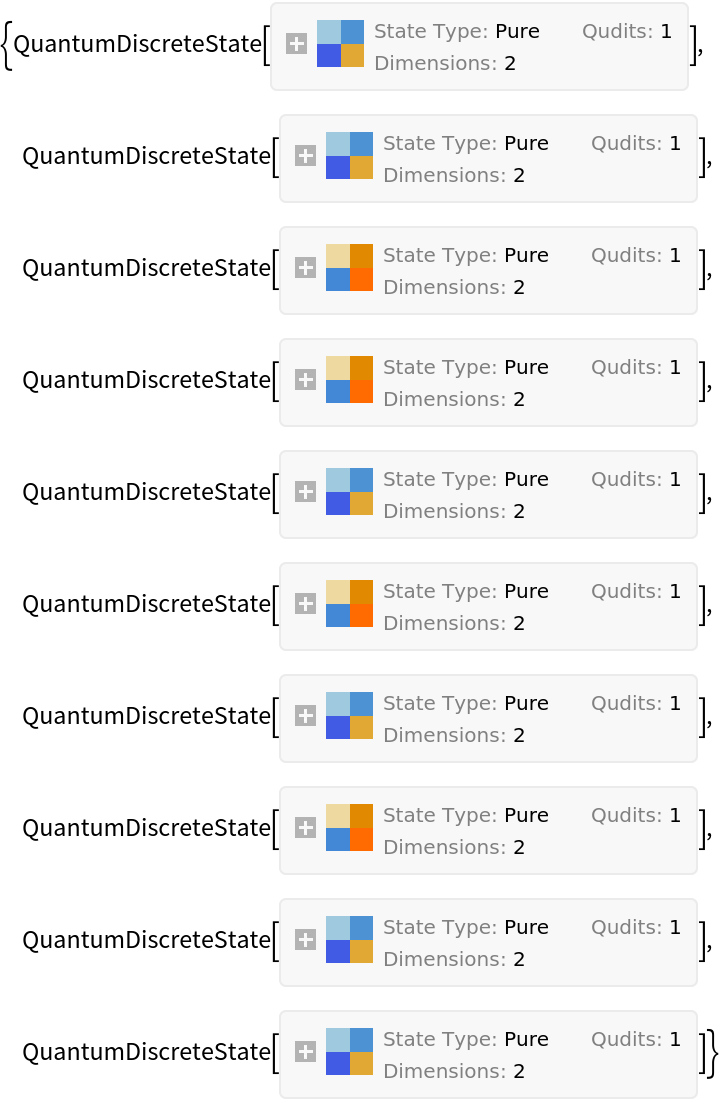Return the symbolic mean of all QuantumDiscreteState objects:

 In:=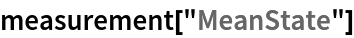Out=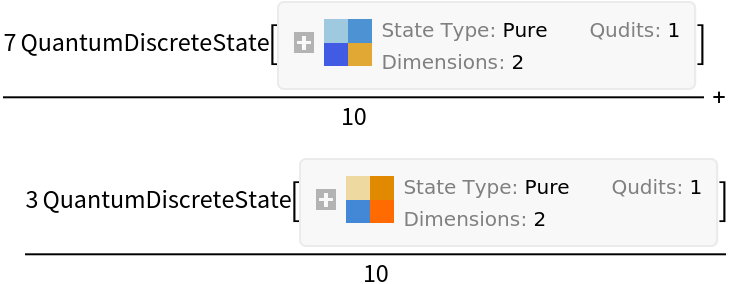Jonathan Gorard

## Version History

• 1.0.0 – 12 April 2021